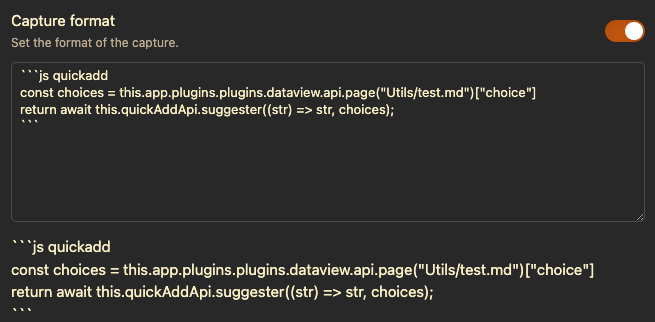let aTexts = [“待办”,“待办”,“灵感”,“消费”,“摘录” ,“留影”,“软件”,“账号”];
let choice = await this.quickAddApi.suggester(aTexts, aTexts);

md可考虑的方式：

dfdf
dkfjdk
dfjkdjf

2, 不能，就直接读取整个md，一行一个配置
dfdf
dkfjdk
dfjkdjf

1 个赞

``````### 任意标题

Choice: 灵感
Choice: 留影
...
Choice: 账号
...
``````

`````````js quickadd
// let aTexts = ["待办 :zap:","待办","灵感","消费","摘录" ,"留影","软件","账号"];

const config_file = app.vault.getAbstractFileByPath("path/to/配置笔记.md");
let lines = await content.split("\n")
.filter(line => line.trim().startsWith("Choice: "))
.map(line => line.split(':').trim());
let choice = await this.quickAddApi.suggester(lines, lines);
return choice;
```
``````

@Probe 你有在ob测试过吗？ THX

``````### 任意标题

Choice:: 灵感
Choice:: 留影
...
Choice:: 账号
...
``````
``````//dv.page()

dv.paragraph(dv.current()['Choice'].join(', '))
````````````const lines = this.app.plugins.plugins.dataview.api.page("0 资料筛选/0筛取规则0.md")["choice"];
let choice = await this.quickAddApi.suggester((str) => str, lines);
``````

``````const lines = this.app.plugins.plugins.dataview.api.page("0 资料筛选/0筛取规则0.md")["choice"];
let choice = await this.quickAddApi.suggester(lines, lines);
``````

md里的相关部分的内容是：

``````### 闪电日记选项列表
choice: 待办
choice: 摘录
...
``````
``````let choice = await this.quickAddApi.suggester((str) => str, lines);
``````

``````let lines = await content.split("\n")
.filter(line => line.trim().startsWith("choice: "))
.map(line => line.split(':').trim());
let choice = await this.quickAddApi.suggester(lines, lines);
``````

``````const lines = this.app.plugins.plugins.dataview.api.page("0 资料筛选/0筛取规则0.md")["choice"];
let choice = await this.quickAddApi.suggester((str) => str,lines);
``````

@iins

^^^^